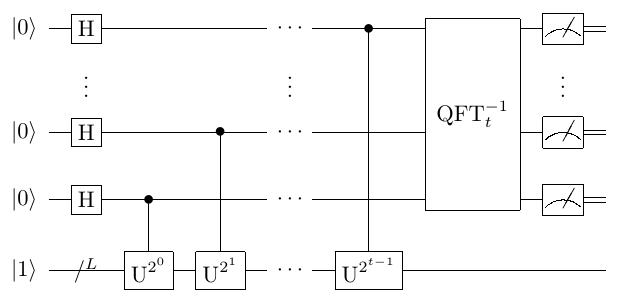# Shor算法¶

Shor算法，又叫质因数分解算法，在破解RSA加密方面有着重要意义。

## 问题背景¶

Shor算法试图解决的极大比特数RSA问题使用经典算法在理论上所需的计算时间和空间资源是近乎无法满足的，它不再只体现了量子计算的相对优势，而是揭示了特定问题上量子计算的不可取代性和绝对优势。

## 算法原理¶

Shor分解算法的具体步骤如下：

1. $$\forall 1<x<N,\ x\in\mathbb{Z}$$
2. $$gcd(x,N)\neq 1$$，结束；
3. $$r$$ 使得 $$x^r mod N≡1$$
4. $$r\ mod\ 2\equiv1$$，回到1取 $$\dot{x}≠x$$
5. $$x^\frac{r}{2}\ mod\ N\equiv-1$$，回到1取 $$\dot{x}≠x$$
6. $$gcd(x^\frac{r}{2}-1,N)gcd(x^\frac{r}{2}+1,N)=N$$

$$f\left(x\right)=x^a\mathrm{\ mod\ N},f\left(a+r\right)=f\left(a\right)$$，求最小的 $$r$$

1. 公式变形所需的前置引理。
2. 构造出可用的模乘量子门操作以迭代完成模指逆元量子态构建。
3. 参考QPE对构造出的模乘求和形式的结果以逆量子傅里叶变换得到模指逆元。

### 前置引理¶

\begin{aligned} \left|u_s\right\rangle\equiv\frac{1}{\sqrt{r}}\Sigma_{k=0}^{r-1}e^{-\frac{2\pi\ iks}{r}}\left|x^k\ mod N\right\rangle,\ x^rmod\ N\equiv1. \end{aligned}

\begin{aligned} \frac{1}{\sqrt{r}}\Sigma_{s=0}^{r-1}e^\frac{2\pi\ iks}{r}\left|u_s\right\rangle=\left|x^k\ modN\right\rangle. \end{aligned}

\begin{aligned} \exists U,U\left|y\right\rangle=\left|xy\ modN\right\rangle,s.t.U\left|u_s\right\rangle=e^\frac{2\pi is}{r}\left|u_s\right\rangle. \end{aligned}

\begin{aligned} \frac{1}{\sqrt{r}}\Sigma_{s=0}^{r-1}\left|u_s\right\rangle=\left|1\right\rangle. \end{aligned}

### 构造模乘量子门¶

\begin{aligned} U^{2^{t-1}z_{t-1}}U^{2^{t-2}z_{t-2}}\cdots U^{2^0z_0}\left|1\right\rangle\approx\left|1\ast x^z\ modN\right\rangle. \end{aligned}

### 求解模指逆元¶

\begin{aligned} (H^{\otimes t}{\otimes I^{\otimes L})(\left|0\right\rangle}^{\otimes t}\left|1\right\rangle_L) =\left|+\right\rangle^{\otimes t}\otimes\left|1\right\rangle_L. \end{aligned}

\begin{split}\begin{aligned} &\prod_{j=1}^{t}\left(C-U^{2^{j-1}}\right)\left(\left|+\right\rangle^{\otimes t}\otimes\left|1\right\rangle_L\right)\\ & =\frac{1}{{\sqrt2}^t}\sum_{j=0}^{2^t-1}\left|j\right\rangle\left|x^j\ modN\right\rangle \\ & =\frac{1}{\sqrt{r2^t}}{\Sigma_{j=0}^{2^t-1}\Sigma}_{s=0}^{r-1}e^\frac{2\pi\ ijs}{r} \left|j\right\rangle\left|u_s\right\rangle=:\left|\psi\right\rangle. \end{aligned}\end{split}

\begin{aligned} ({\rm QFT}^{-1}\otimes I^{\otimes L})\left|\psi\right\rangle=\frac{1}{\sqrt r}\Sigma_{s=0}^{r-1} \left|\frac{2^ts}{r}\right\rangle\left|u_s\right\rangle. \end{aligned}

## 量子线路图与参考代码¶

Shor算法的量子线路图如下所示std::pair<bool, std::pair<int,int>> Shor_factorization(int target);


#include "QPanda.h"
using namespace QPanda;

int main(void)
{
int N = 15, factor_1, factor_2;
auto p = Shor_factorization(N);
cout << boolalpha << p.first << "," << p.second.first << "," << p.second.second << endl;

return 0;
}


$$15$$ 的质因子分解结果应该是 $$15=3*5$$ ，所以应当返还算法成功标志和两个质因子 $$3$$$$5$$

true, 3, 5